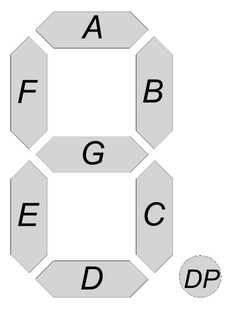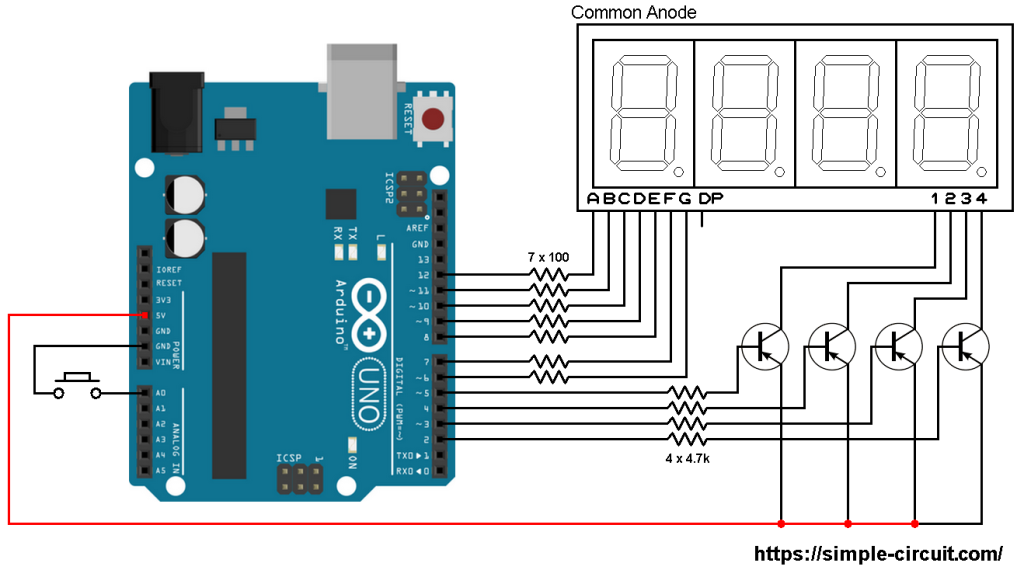# Interfacing Arduino with 7-segment display | 4-Digit counter example

This post shows how to interface Arduino UNO board with 7-segment display in order to build a simple 4-digit counter which counts from 0 to 9999. A push button connected to Arduino is used to increment the displayed number.

There are two types of the seven-segment displays: common anode and common cathode.
In the common anode type all the 7 LED anode terminals are connected together whereas in the common cathode all cathode terminals are connected together.

The common terminal is connected to +VCC (+5V, +3.3V …) or GND (0V) depending on the type of the 7-segment display (common anode or common cathode respectively).

Basically for each 7-segment digit there are 8 pins: one for the common terminal (anode or cathode) and 7 pins for the 7 segments (A, B, C, D, E, F and G). Another pin may be used for the decimal point (DP).In multi-digit 7-segment display (for example 4-digit) all pins of the same segment are connected together (segment A of digit 1 with segment A of digit 2 …), and each digit has its common pin alone. This is called multiplexing technique. This technique minimizes number of pins used.

So for a 4-digit display we’ll have 7 pins of the 7 segments, 4 pins of the 4 digits (common terminals) and 1 pin for the decimal point (DP) which means a total of 12 pins.

Hardware Required:

• Arduino UNO board
• 4-Digit common anode 7-segment display
• 4 x PNP transistor (2SA1015, 2S9015, 2N3906 …)
• 7 x 100 ohm resistor
• 4 x 4.7k ohm resistor
• Push button
• Jumper wires

Interfacing Arduino with 7-segment display circuit:
Example circuit schematic diagram is shown below.The push button which is connected to Arduino A0 pin is used to increment the displayed number.
A common anode 7-segment display is used in this example.

In the circuit there are 4 transistors of the type PNP, the collector of each transistor is connected to common anode pin of 1 digit. That means each transistor supplies one digit segments.
The 4 transistors are used to supply the display LEDs with sufficient current because Arduino microcontroller (ATmega328P) may not be able to do that (maximum output current is 40mA).
Each transistor emitter pin is connected to +5V that comes from the Arduino board and each transistor base is connected to the Arduino through 4.7k resistor.

Each 100 ohm resistor is used for limiting the current that passes through the segment LED.

Interfacing Arduino with 7-segment display code:
Example Arduino code is below.

Since the 4 digits are multiplexed we need to refresh the display very quickly (display one digit at a time, others are off). For that I used Timer1 module interrupt with the following configuration:

With the above configuration Timer1 module overflows every 4096 microseconds (4096 = 65536/16) which is a good refresh period.

Note that Timer1 module is 16-bit timer, prescaler = 1 (TCCR1B = 1) and Arduino UNO clock = 16MHz.

The following video shows a simple protoboard circuit of the example:

and the video below shows Proteus simulation (simulation circuit is not the same as real hardware circuit, example circuit diagram is shown above):

Arduino 7-segment display counter

1.Anjana says:

how this can turn in to a 5 digit display

2.wgc says:

count++; // increment ‘count’ by 1
if(count > 9999)
count = 0;
delay(200);

if(count > 9999)

1.Mr.Naing says:

Thank you I got it fixed as you said.

3.Kenjutsu says:

Good day,

How was the base resistor value of 4.7k calculated?

4.Curie Gupta says:

Hi,
Why are we taking pnp transistor and not npn?

1.Simple Projects says:

Basically, choosing of transistor type NPN or PNP depends on circuit design. In this project we’ve a common anode 7-segment display. This means each common pin have to be connected to +5V in order to turn on its related digit.
The NPN transistor type allows current flows from collector to emitter when base-emitter voltage is greater than threshold voltage (about 0.7V).
The PNP transistor allows current flows from collector to emitter when base-emitter voltage is lower than threshold voltage (about -0.7V).
In this project if we use a NPN type then its collector will be connected to +5V and its emitter to the common pin of 7-segment display. Normally, the NPN transistor turns on when the Arduino outputs logic high (+5V). If there’s a 5V at base and 5V at emitter how will the transistor turn on?
You can use NPN type but the 7-segment display will not be supplied correctly with 5V (about 4 Volts) which yields low brightness.
If you have a common cathode 7-segment display then you should use NPN type.

5.Karanbir Soin says:

In this example to show 2056 on the display we need <= 2055 button presses.
Can another switch be added to select the digits(ones tens hundreds and thousands and the increment the number on each digit. The number will increase upto 9 and roll over to 0 but will not go to next digit.

1.Simple Projects says:

Yes you can do that, but you’ve to make some modifications to the code!

1.Joz says:

Any suggestions for setting a prescaler for 2048us and 1024us in use as PWM?

6.Vainavi says:

Hi, can anyone tell me how to take any number input from the user and display that on the 7 segment 4 number display? I used serial.parseint(), but its not showing the digits in the tens, hundredths and the thousandths place.

7.Aaron says:

If you are using the example code from this project, and using serial.parseint() to supply the value for ‘count’, it should work. You should be able to read and display values from 0 to 9999. If you share your code, someone might be able to identify why it isn’t functioning as expected.

8.Jack says:

This code can’t be verified as the disp_off(); hasn’t been defined. I’d offer up more information but I’m not entirely sure as to what it means.

1.Jack says:

I have gotten past this now but I am struggling to get the button to increment the counter. I have adjusted the code for a common cathode display, with NPN and grounding instead of pulling up to 5V but I’m still missing something to get the button to have any effect.

This site uses Akismet to reduce spam. Learn how your comment data is processed.